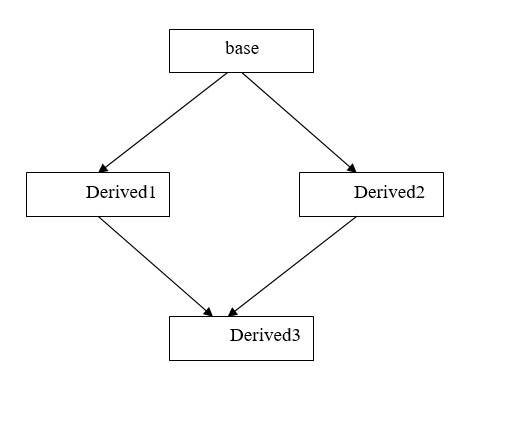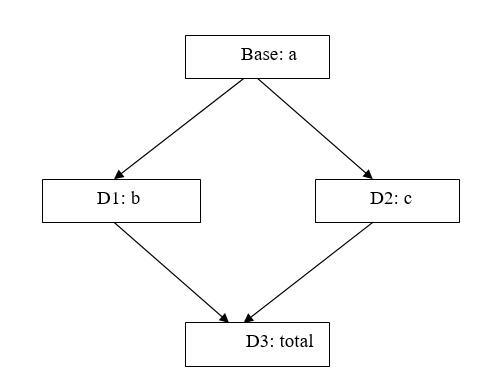# C++: Inheritance Virtual base class (Multi-Path Inheritance)

Virtual base class (Multi-Path Inheritance)

When a class inherits the properties of another class two or more times through two or more different paths it is known as multipath inheritance.

The properties are inherited more than once, so more memory is consumed and ambiguity (doubt) gets created, in order to avoid this extra consumption of memory and ambiguity, we declare the base class as virtual while giving the definition of the derived class.

On declaring the base class as virtual the properties of the base class are inherited only once and hence no extra memory is consumed and the problem of ambiguity automatically gets solved.Syntax:

class base
{
};
class derived1:public virtual / virtual public base
{
};
class derived2:public virtual/ virtual public base
{
};
class derived3:public derived1,public derived2
{
};

Example:1We want to calculate the sum of all the data elements (“a” of class Base, “b” of class D1 and “c” of class D2) and store them in “total” of class D3.

```#include<iostream>
#include<conio.h>
using namespace std;
class base
{
public:
int a;
};
class D1:public base
{
public:
int b;
};
class D2:public base
{
public:
int c;
};
class D3:public D1,public D2
{
public:
int total;
};

int main()
{
D3 ob;
ob.a=10; // error
//this is ambiguous , which a? from D1 or D2 as
// D3 inherits from both D1 and D2
ob.b=20;
ob.c=30;
ob.total=ob.a+ob.b+ob.c;
cout<<"total "<<ob.total<<endl;
return(0);
}
```

Modified Program

```//virtual base  inheritance
#include<iostream>
#include<conio.h>
using namespace std;
class base
{
public:
int a;
};
class D1:virtual public base
{
public:
int b;
};
class D2:virtual public base
{
public:
int c;
};
class D3:public D1,public D2
{
public:
int total;
};
int main()
{
D3 ob;
ob.a=10; // no error
//this is now  unambiguous as only one copy is inherited
ob.b=20;
ob.c=30;
ob.total=ob.a+ob.b+ob.c;
cout<<"a = "<<ob.a<<endl;
cout<<"b = "<<ob.b<<endl;
cout<<"c = "<<ob.c<<endl;
cout<<"total "<<ob.total<<endl;
return(0);
}
```

Output:

a = 10
b = 20
c = 30
total 60